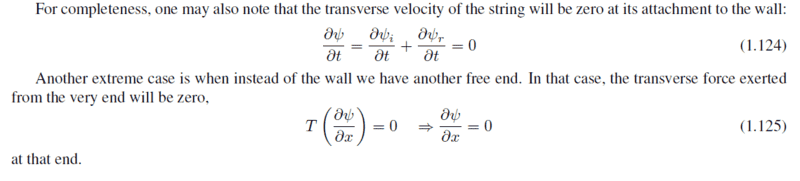# Question about a stretched string's motion at the free end1. I don't know why ∂Ψ/∂x should be zero in (1.125). Shouldn't the tension be zero at the very last end?
2. I don't know why we can apply the results of the wave equation at the free end because we assumed there are tension both sides of the string segment during the derivation of the wave equation.

#### Attachments

Chestermiller
Mentor
I don’t think they are assuming that the end of the string is totally unconstrained. I think they are assuming that the string is still under tension axially, but it is free to move laterally. Imagine the end of the string attached to a small ring, with a stationary frictionless rod running vertically through the ring. So the ring can move up and down on the rod, but not left and right.

•Clara Chung
For the second question, the derivation of the wave equation was done by considering all string elements that have other string elements on either side. The imposition of boundary conditions does not violate this assumption, because boundary conditions only apply to those string elements that are on the boundary, i.e., to those string elements that do not have other string elements on either side.

•Clara Chung
Thanks for the answer. In this case, does it mean I can't use the formula ∂Ψ/∂x=-1/c ∂Ψ/∂t, to get the vertical velocity =0 of the segment of the end of the string?
For the second question, the derivation of the wave equation was done by considering all string elements that have other string elements on either side. The imposition of boundary conditions does not violate this assumption, because boundary conditions only apply to those string elements that are on the boundary, i.e., to those string elements that do not have other string elements on either side.

Chestermiller
Mentor
Thanks for the answer. In this case, does it mean I can't use the formula ∂Ψ/∂x=-1/c ∂Ψ/∂t, to get the vertical velocity =0 of the segment of the end of the string?
There is no significant movement of material along the axis of the string. All the movement is assumed to be normal to the axis, and the material velocity is just ##\partial \psi/\partial t##. The wave velocity is c. At the laterally unconstrained end, ##\partial \psi/\partial x=0##, but not ##\partial \psi/\partial t##.

There is no significant movement of material along the axis of the string. All the movement is assumed to be normal to the axis, and the material velocity is just ##\partial \psi/\partial t##. The wave velocity is c. At the laterally unconstrained end, ##\partial \psi/\partial x=0##, but not ##\partial \psi/\partial t##.
I know that the wave velocity is c. However if I substitute ∂ψ/∂x=0 into ∂Ψ/∂x=-1/c ∂Ψ/∂t to find the normal velocity of the segment of the string at the free end, ∂Ψ/∂t becomes zero. This means the segment is not moving at all at the free end which is a contradiction.

Chestermiller
Mentor
I know that the wave velocity is c. However if I substitute ∂ψ/∂x=0 into ∂Ψ/∂x=-1/c ∂Ψ/∂t to find the normal velocity of the segment of the string at the free end, ∂Ψ/∂t becomes zero. This means the segment is not moving at all at the free end which is a contradiction.
Where did the equation ##\frac{\partial \psi}{\partial t}=-c\frac{\partial \psi}{\partial x}## come from?

Where did the equation ##\frac{\partial \psi}{\partial t}=-c\frac{\partial \psi}{\partial x}## come from?
It comes from the wave equation if ψ = f(kx-wt). Does it mean I can't use the equation because ψ = f(kx-wt) + g(kx+wt)?

Chestermiller
Mentor
It comes from the wave equation if ψ = f(kx-wt). Does it mean I can't use the equation because ψ = f(kx-wt) + g(kx+wt)?
Please show how it derives from that ewuation.